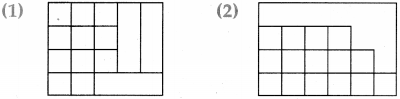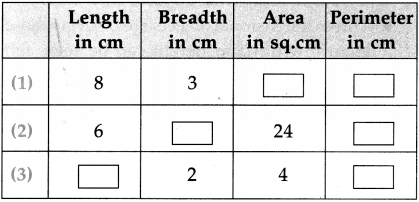Balbharti Maharashtra Board Class 5 Maths Solutions Chapter 12 Perimeter and Area Problem Set 50 Textbook Exercise Important Questions and Answers.

## Maharashtra State Board Class 5 Maths Solutions Chapter 12 Perimeter and Area Problem Set 50

Question 1.
The length of the side of each square is given below. Find its area.

(1) 12 metres
Solution:
Area of a square
= side x side
= 12 x 12(2) 6 cm
Solution:
Area of a square
= side x side
= 6 x 6
= 36 sq.cm.

(3) 25 metres
Solution:
Area of a square
= side x side
= 25 x 25
= 625 sq.m.

(4) 18 cm
Solution:
Area of a square
= side x side
= 18 x 18
= 324 sq.cm.Question 2.
If the cost of 1 sq m of a plot of land is 900 rupees, find the total cost of a plot of land that is 25 m long and 20 m broad.
Solution:
Area of the rectangular plot
= 25 x 20
= 500 sq.m.

Cost of the plot of land
= Area of the plot x rate
= 500 x 900
= 4,50,000 rupees

Question 3.
The side of a square is 4 cm. The length of a rectangle is 8 cm and its width is 2 cm. Find the perimeter and area of both figures.
Solution:
Perimeter of a square = 4 x side
= 4 x 4
= 16 cm

Area of a square = side x side
= 4 x 4
= 16 sq.cm.

Perimeter of a rectangle
= 2 x length + 2 x breadth
= 2 x 8 + 2 x 2
= 16 + 4
= 20 cm

Area of a rectangle
= 8 x 2
= 16 sq.cm.Question 4.
What will be the labour cost of laying the floor of an assembly hall that is 16 m long and 12 m wide if the cost of laying 1 sq m is 80 rupees?
Solution:
Area of rectangular floor
= 16 x 12
= 192 sq.cm.

The cost of laying 1 sq.m, is 80 rupees.
Hence, the cost of laying 192 sq.m.
= 192 x 80
= 15,360 rupees.
∴ ₹ 15,360

Question 5.
The picture alongside shows some squares. Find out how many squares with the same measures will fit in the empty space in the figure.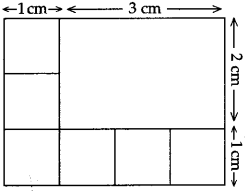Solution:
length of the empty space = 4 – 1 = 3 cm
breadth of the empty space = 3 – 1 = 2 cm
square in empty space
= 3 x 2 = 6 sq.cm.

∴ 6 squares will fit in the empty space in the figureQuestion 6.
Divide the figure given alongside into four parts in such a way that the area and shape of each part is the same. Colour the parts with different colours.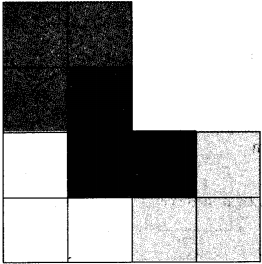Fair and square

As shown in the figure alongside, a square plot of land owned by the government contains four houses and a well right in the centre. The government has to divide the houses and the land between four poor persons according to the following conditions.
(1) Each person must get only one house.
(2) The shape and area of the land must be the same.
(3) Each person must be able to use the well without trespassing on any one else’s land.

Show the appropriate divisions in four different colours.

Activity
Using a graph paper, find out the area of different rectangles and squares.

Question 1.
15 cm
Solution:
Area of a square
= side x side
= 15 x 15
= 225 sq.cm.Question 2.
21 cm
Solution:
Area of a square = side x side
= 21 x 21
= 441 sq.cm.

Solve the following:

Question 1.
The side of a square hall is of length 8 m. If it is tiled with a tile of length 4 m and breadth 2 m., how many tiles will be required?
Solution:
Area of the square hall
= side x side
= 8 x 8
= 64 sq.m.

Area of 1 rectangular tile
= 4 x 2

∴ 8 sq.m.

For 8 sq.m., 1 tile is required,
but for 64 sq.m = $$\frac{64}{8}$$ = 8 tiles required

∴ 8 tiles requiredQuestion 2.
Perimeter of a square is 16 cm. What is the length of each side? What is the area of the square?
Solution:
Perimeter of square = 4 x side
16 = 4 x side

∴ side of a square = $$\frac{16}{4}$$ = 4 cm
Area of the square
= side x side
= 4 x 4
= 16 sq.cm

∴ Side of square is 4 cm and area of the sqaure is 16 sq.cm

Question 3.
Write the perimeter of each figure in the box given below it.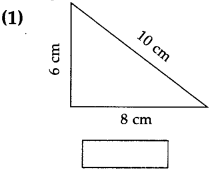24 cm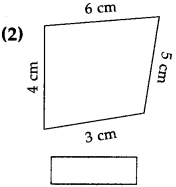18 cm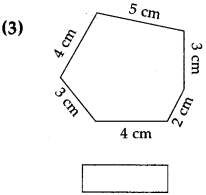21 cm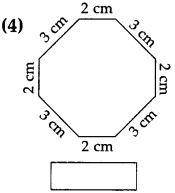20 cmQuestion 4.
Two squares of side 2 cm is cut out of two corners of a larger square with side 5 cm (see the figure). What will be the perimeter of the remaining shape?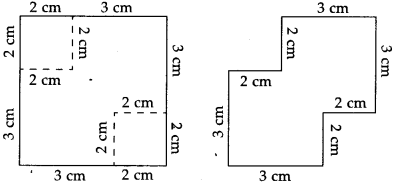20 cm

Question 5.
Match the columns ‘A’ and ‘B’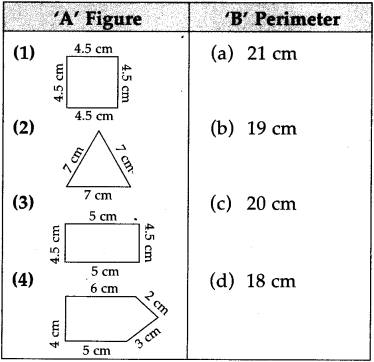(1- d),
(2- a),
(3 – b),
(4 – c)Question 6.
Solve the following word problems:
(1) What is the perimeter of a rectangle having length 9 cm and its breadth 6 cm?
(2) The sides of a rectangular field are having length 150 m and breadth 100 m. Find the perimeter of field.
(3) If each side of a square is 8 cm then what is the perimeter of the square?
(4) A rectangular garden of length 650 m and breadth 350 m. Mohan makes four rounds daily. How many kilometres does he walk everyday?(5) One square field is having one side of it is of 225 m, Soham makes 6 rounds of the square field daily. How much distance is covered by him? Write it in km and m.
(6) A rectangular field whose length is 58 m and breadth is 32 m. Fencing the field by 4 rounds with a wire, what length of wire is required? If the cost of 1 m wire is ? 75, then what is the expenditure of fencing the field?
(7) A length of a rectangular classroom is 8 m and its breadth is 5 m. A wooden strip is to be fitted along the four walls to hang charts and pictures. What is the length of the wooden strip required?
(8) The side of a square table is 1.5 m. To fit a strip of tin sheet around the table, how many metres of strip is required?
(9) What is the perimeter of the triangle whose sides are 13.8 cm, 17.6 cm and 10.6 cm?
(10) The sides of some squares are given below. Find their areas.
(i) 11 cm (ii) 23 cm (iii) 9 cm(11) Find the perimeter and area of the following:
(i) square of side 6 cm
(ii) Rectangle: length 12 cm and breadth 6 cm
(12) A rectangular land is having its length 25 m and breadth 16 m. If the cost of 1 sq.m, land is ? 1,500, then what will be cost of land?
(13) The side of a square plot is 10 metre. It is tiled at the rate of 50 rupees per sq.m. What will be cost of tiling the floor?
(14) A wall of length 25 m and breadth 12 m. It is painted at the rate of 60 rupees per sq.m. What will be cost of painting the wall?
(1) 30 cm
(2) 500 m
(3) 32 cm
(4) 8 km
(5) 5 km 400 m
(6) ₹ 54,000
(7) 26 m
(8) 6 m
(9) 42 cm

(10) (i) 121 sq. cm
(ii) 529 sq.cm
(iii) 81sq.cm.

(11) (i) Perimeter = 24 cm, Area = 36 sq.cm,
(ii) Perimeter = 36 cm, Area = 72 sq. cm

(12) 6,00,000 rupees
(13) 5,000 rupees
(14) t 18,000Question 7.
Look at the figures on the sheet of graph paper. Measure their sides with the help of the lines on the graph paper. Write the perimeter of each in the right box.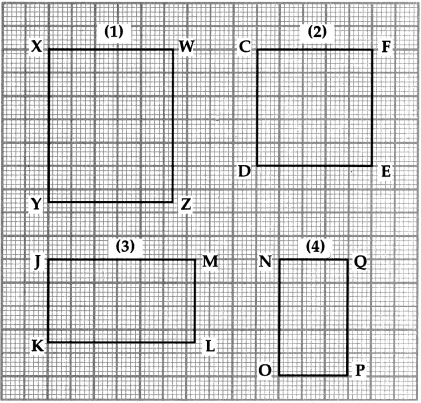Perimeter of Rectangle
(1) XYZW = [ ] cm
(2) CDEF = [ ] cm
(3) JKLM = [ ] cm
(4) NOPQ = [ ] cm
(1) 12 cm
(2) 10 cm
(3) 10 cm
(4) 8 cmQuestion 8.
Find the area of the. following figures. (All small squares are having side 1 cm)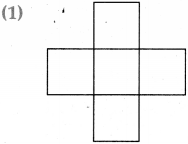5 sq.cm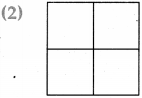4 sq.cm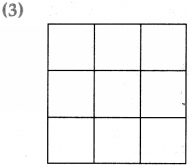9 sq.cm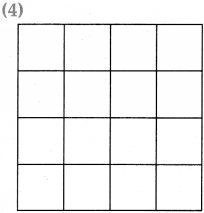16 sq.cm.Question 9.
The pictures below shows some squares. Find out how many squares with the same measures will fit in the empty space in the figures.